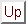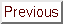Next: Generating . Up: Unconstrained Optimization Previous: An estimation of the   Contents

# The secondary Trust-Region subproblem

The material of this chapter is based on the following reference: [Pow00].
We seek an approximation to the solution, of the maximization problem:subject toThe following maximization problem is equivalent (after a translation) and will be discussed in the chapter: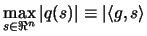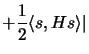(5.1) subject to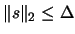We will indifferently use the term, polynomial, quadratic or model. The trust region'' is defined by the set of all points which respect the constraint.
Further, the shape of the trust region allowsto be replaced by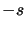, it's thus equivalent to consider the computation(5.2) subject toNow, ifand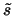are the values that maximize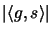and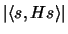, respectively, subject to, thenmay be an adequate solution of the problem 5.1, if it is the choice between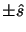and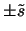that gives the largest value of the objective function of the problem. Indeed, for every feasible, including the exact solution of the present computation, we find the elementary bound(5.3)

It follows that the proposed choice ofgives a value ofthat is, at least, half of the optimal value. Now,is the vector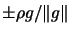, whileis an eigenvector of an eigenvalue of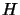of largest modulus, which would be too expensive to compute. We will now discuss how to generate. We will use a method inspired by the power method for obtaining large eigenvalues.
Because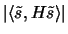is large only if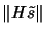is substantial, the technique begins by finding a column of,say, that has the greatest Euclidean norm. Hence lettingbe the columns of the symmetric matrix, we deduce the bound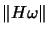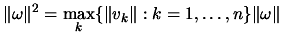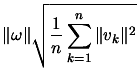(5.4)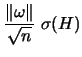(5.5)

Where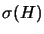is the spectral radius of. It may be disastrous, however to setto a multiple of, because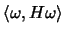is zero in the case: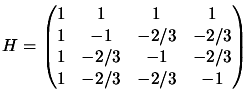(5.6)

Therefore, the algorithm picksfrom the two dimensional linear subspace of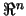that is spanned byand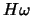. Specifically,has the form, where the ratiois computed to maximize the expression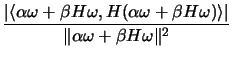(5.7)

which determines the direction of. Then the length ofis defined by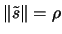, the sign of, being unimportant.

SubsectionsNext: Generating . Up: Unconstrained Optimization Previous: An estimation of the   Contents
Frank Vanden Berghen 2004-04-19